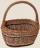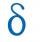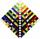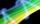Saving per cents

The first day I save 1 cent and every next day cent more.
How many I saved per year (365 days)?

Result

x =  667.95 eur

Solution:Leave us a comment of this math problem and its solution (i.e. if it is still somewhat unclear...):

Showing 1 comment:Math student
why thoNext similar math problems:

1. SeatsSeats in the sport hall are organized so that each subsequent row has five more seats. First has 10 seats. How many seats are: a) in the eighth row b) in the eighteenth row
2. Apples in basketsDetermine how many apples are in baskets when in the first basket are 4 apples, and in any other is 29 apples more than the previous, and we have eight baskets.
3. Sequence 2Write the first 5 members of an arithmetic sequence a11=-14, d=-1
4. AP - simpleDetermine the first nine elements of sequence if a10 = -1 and d = 4
5. SequenceWrite the first 7 members of an arithmetic sequence: a1=-3, d=6.
6. Sequence 3Write the first 5 members of an arithmetic sequence: a4=-35, a11=-105.
7. SequenceWrite the first 6 members of these sequence: a1 = 5 a2 = 7 an+2 = an+1 +2 an
8. Sum of membersWhat is the sum of the first two members of the aritmetic progression if d = -4.3 and a3 = 7.5?
9. Consecutive numbersSum of ten consecutive numbers is 105. Determine these numbers (write first and last).
10. 6 termsFind the first six terms of the sequence. a1 = 7, an = an-1 + 6
11. AP - simpleFind the first ten members of the sequence if a11 = 132, d = 3.
12. Difference APCalculate the difference of arithmetic progression if the sum of its first 19 members Sn = 8075 and the first member is a1 = 20
13. Theorem proveWe want to prove the sentence: If the natural number n is divisible by six, then n is divisible by three. From what assumption we started?
14. SeriesYour task is express the sum of the following arithmetic series for n = 14: S(n) = 11 + 13 + 15 + 17 + ... + 2n+9 + 2n+11
15. AS sequenceIn an arithmetic sequence is given the difference d = -3 and a71 = 455. a) Determine the value of a62 b) Determine the sum of 71 members.
16. SequenceBetween numbers 1 and 53 insert n members of the arithmetic sequence that its sum is 702.
17. VectorsFor vector w is true: w = 2u-5v. Determine coordinates of vector w if u=(3, -1), v=(12, -10)